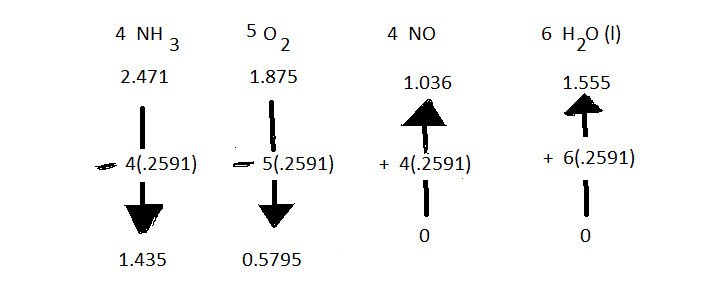The following problem, which will be solved here using an "arrow diagram," was posted in a question at Physics Forums: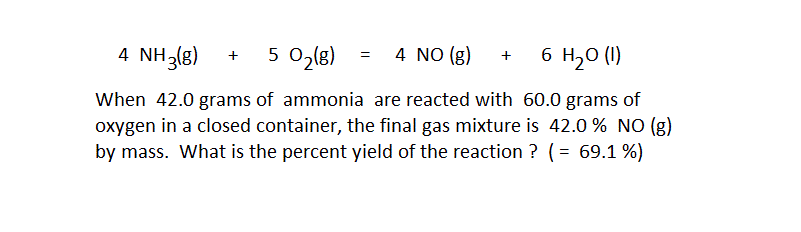The solution to this problem must be based on the given fact that the final reaction mixture, excluding only the water formed by the reaction, is 42% NO (g) by mass. To set up the preliminary arrow diagram, 42.0 grams of ammonia and 60.0 grams of oxygen are changed to moles by conventional unit conversion calculations. The preliminary arrow diagram then looks like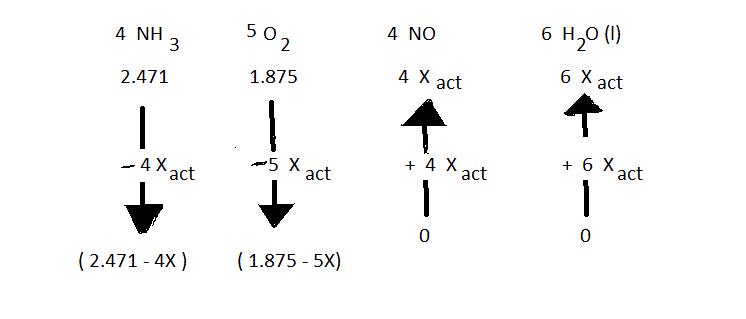ANY question about the reaction should be answerable if all positions in the arrow diagram can be specified with numbers. This requires the calculation of Xactual as a number. In this case, the value of Xact is calculated from the fact that the final mixture of gases is 42 % NO(g) by mass. As an equation

grams NO(g) formed = 0.42 (102 Total grams - grams of water formed )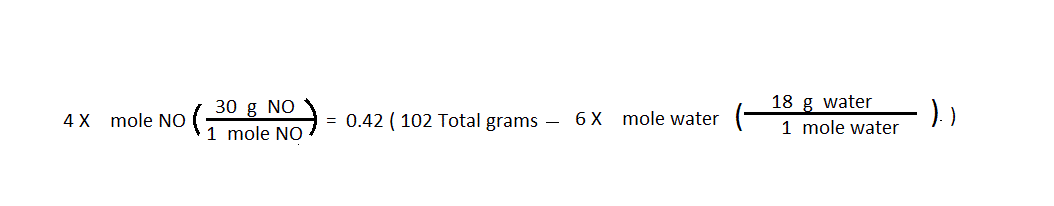Solving this equation gives Xact = 0.2591. There is no unit because this value applies to the entire reaction.

The value of Xact should be used to answer the question in the most direct was possible. The percent yield of a reaction can be calculated as the ratio of Xact to X100 multiplied by 100: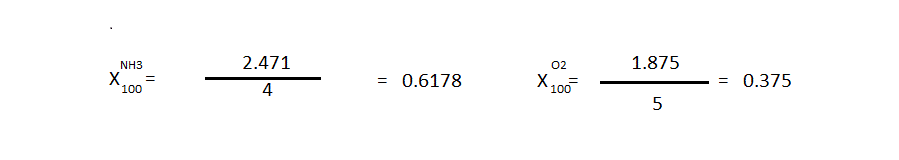The SMALLER of the calculated values of X100 is the real value of X100. Then, with both Xact and X100 being known, the percent yield of the reaction is calculated: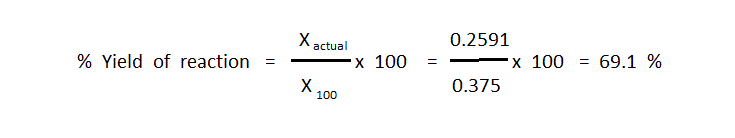Once the percent can be calculated, the problem has been solved. There is no need in this problem to complete the arrow diagram with numbers. That would be needed in some problems though, so using the value of Xact the arrow diagram could be completed as follows: